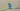# 箭头函数与常规函数的 5 个不同

``````// 函数声明
function greet(who) {
return `Hello, \${who}!`;
}``````
``````// 函数表达式
const greet = function (who) {
return `Hello, \${who}`;
};``````

``````const greet = (who) => {
return `Hello, \${who}!`;
};``````

## `this` 的值

### 常规函数

``````function myFunction() {
console.log(this);
}

// 简单引用
myFunction(); // 输出全局对象(window)``````

``````const myObject = {
method() {
console.log(this);
},
};
// 方法引用
myObject.method(); // 输出 "myObject"``````

``````function myFunction() {
console.log(this);
}

const myContext = { value: 'A' };

myFunction.call(myContext); // 输出 { value: 'A' }
myFunction.apply(myContext); // 输出 { value: 'A' }``````

``````function MyFunction() {
console.log(this);
}

new MyFunction(); // 输出一个 MyFunction 的实例``````

### 箭头函数

``````const myObject = {
myMethod(items) {
console.log(this); // 输出 "myObject"    const callback = () => {
console.log(this); // 输出 "myObject"    };
items.forEach(callback);
},
};

myObject.myMethod([1, 2, 3]);``````

`this` 绑定解析是箭头函数一个巨棒的功能。当在方法内部使用回调函数时可以确认箭头函数不声明它自己的 `this`：不再需要使用 `const self = this``callback.bind(this)` 之类的变通技巧。

## 构造函数

### 常规函数

``````function Car(color) {
this.color = color;
}

const redCar = new Car('red');
redCar instanceof Car; // => true``````

`Car` 是一个常规函数，并且使用 `new` 关键字，它创建属于 `Car` 类型的新实例。

### 箭头函数

``````const Car = (color) => {
this.color = color;
};

const redCar = new Car('red');
// TypeError: Car is not a constructor``````

## arguments 对象

### 常规函数

``````function myFunction() {
console.log(arguments);
}

myFunction('a', 'b'); // 输出 { 0: 'a', 1: 'b'}``````

### 箭头函数

``````function myRegularFunction() {
const myArrowFunction = () => {
console.log(arguments);
};

myArrowFunction('c', 'd');
}

myRegularFunction('a', 'b');
// 输出 { 0: 'a', 1: 'b' }``````

``````function myRegularFunction() {
const myArrowFunction = (...args) => {    console.log(args);  };
myArrowFunction('c', 'd');
}

myRegularFunction('a', 'b');
// 输出 { 0: 'c', 1: 'd' }``````

`...args` 扩展参数聚合箭头函数执行时参数：`{ 0: 'c', 1: 'd' }`

## 内部返回 return

### 常规函数

``````function myFunction() {
return 42;
}

myFunction(); // => 42``````

``````function myEmptyFunction() {
42;
}

function myEmptyFunction2() {
42;
return;
}

myEmptyFunction(); // => undefined
myEmptyFunction2(); // => undefined``````

### 箭头函数

``````const increment = (num) => num + 1;

increment(41); // => 42``````

`increment()` 箭头函数仅包含一个表达式 `num + 1`。箭头函数不需要使用 `return` 关键字直接返回该表达式。

## 方法

### 常规函数

``````class Hero {
constructor(heroName) {
this.heroName = heroName;
}

logName() {    console.log(this.heroName);  }}

const batman = new Hero('Batman');``````

``````setTimeout(batman.logName, 1000);
// after 1 second logs "undefined"``````

1 秒后，`undefined` 在控制台中被打印出来。`setTimeout` 简单引用 `logName`（这时 `this` 是全局对象）。

``````setTimeout(batman.logName.bind(batman), 1000);
// after 1 second logs "Batman"``````

`batman.logName.bind(batman)` 绑定 `this``batman` 实例。现在可以确定该方法没有失去上下文。

### 箭头函数

``````class Hero {
constructor(heroName) {
this.heroName = heroName;
}

logName = () => {    console.log(this.heroName);  };}

const batman = new Hero('Batman');``````

``````setTimeout(batman.logName, 1000);
// after 1 second logs "Batman"``````

## 总结

`arguments` 对象包含了常规函数中的参数列表。而箭头函数则相反，不定义参数（但你可以使用扩展参数 `...args` 轻松访问箭头函数的参数）。